# Smart Coffee Table with Nano and HX711

This smart coffee table illuminates your environment according to the weight of your drink.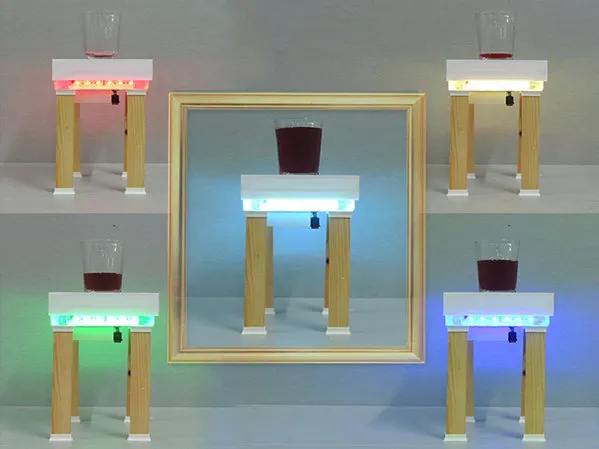## Things used in this project

### Hardware componentsArduino Nano R3
×1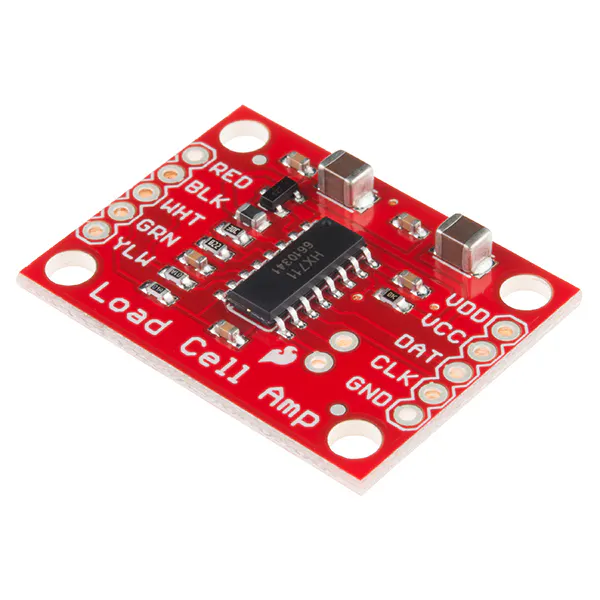SparkFun Load Cell Amplifier - HX711
×1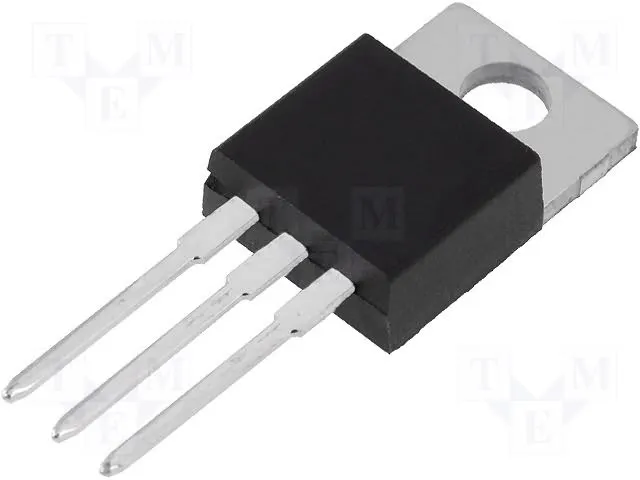Linear Regulator (7805)
×1Resistor 10k ohm
×1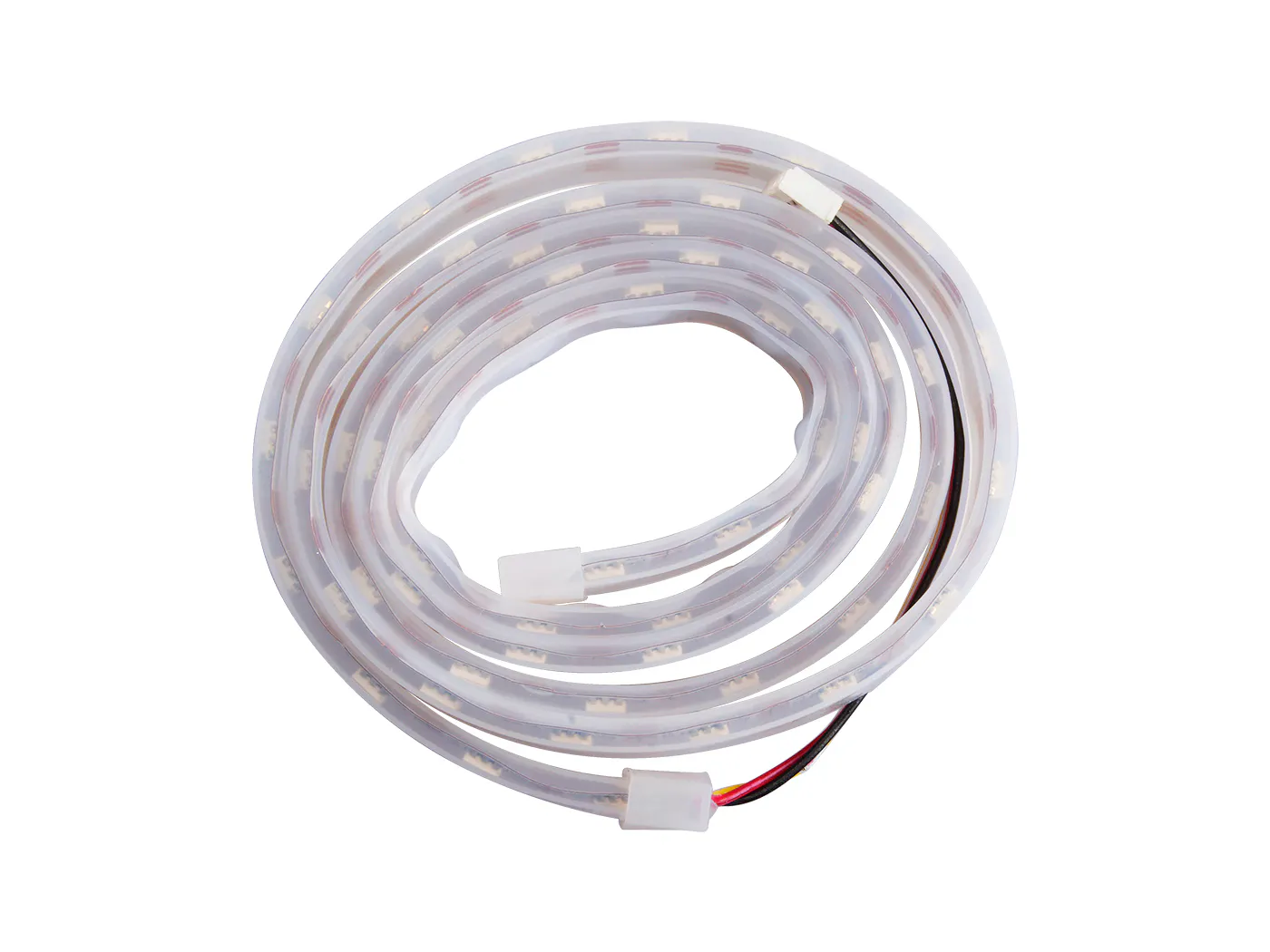Seeed Grove - WS2813 RGB LED Strip Waterproof - 60 LED/m - 1m
×1
 BD 135 Transistor
×1

### Hand tools and fabrication machinesSoldering iron (generic)3D Printer (generic)

## Schematics

### hx71112222_kb1dYR9i2M.png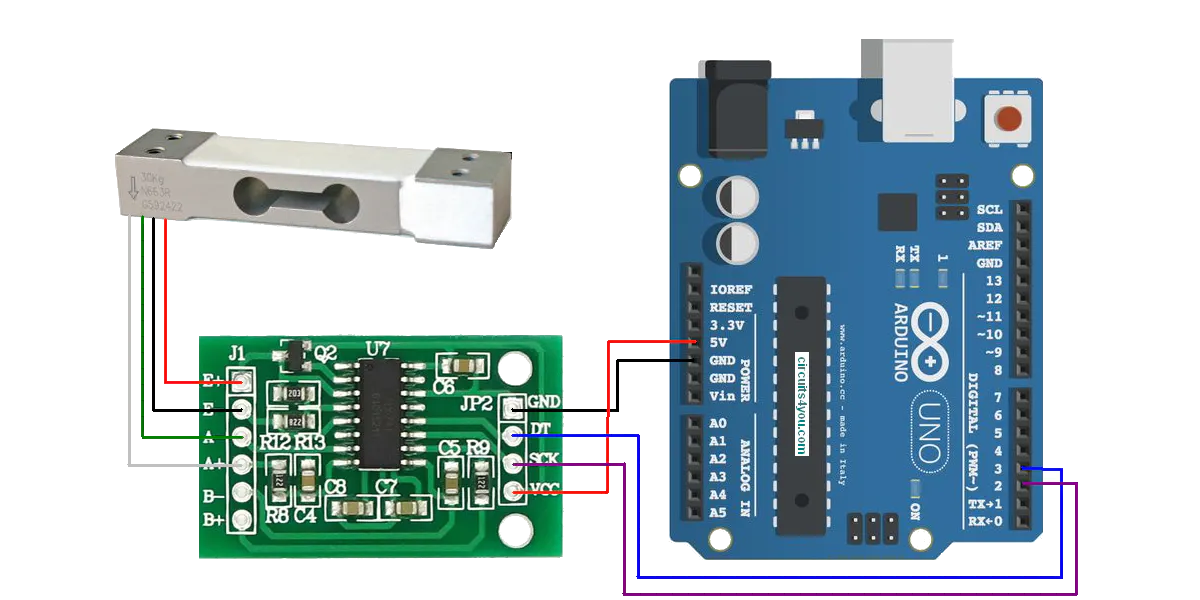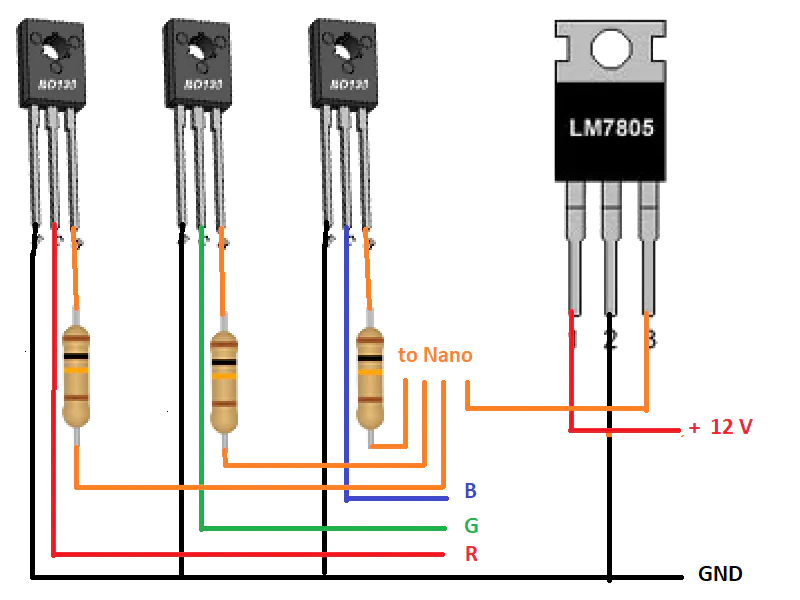## Code

### HX711_full_example.ino

Arduino
```/**
*
* HX711 library for Arduino - example file
* https://github.com/bogde/HX711
*
* (c) 2018 Bogdan Necula
*
**/
#include "HX711.h"

// HX711 circuit wiring

HX711 scale;

void setup() {
Serial.begin(38400);
Serial.println("HX711 Demo");
pinMode(9,OUTPUT);
pinMode(10,OUTPUT);
pinMode(11,OUTPUT);

Serial.println("Initializing the scale");

// Initialize library with data output pin, clock input pin and gain factor.
// Channel selection is made by passing the appropriate gain:
// - With a gain factor of 64 or 128, channel A is selected
// - With a gain factor of 32, channel B is selected
// By omitting the gain factor parameter, the library
// default "128" (Channel A) is used here.

Serial.println("Before setting up the scale:");

Serial.print("get value: \t\t");
Serial.println(scale.get_value(5));		// print the average of 5 readings from the ADC minus the tare weight (not set yet)

Serial.print("get units: \t\t");
Serial.println(scale.get_units(5), 1);	// print the average of 5 readings from the ADC minus tare weight (not set) divided
// by the SCALE parameter (not set yet)

scale.set_scale(2280.f);                      // this value is obtained by calibrating the scale with known weights; see the README for details
scale.tare();				        // reset the scale to 0

Serial.println("After setting up the scale:");

Serial.print("get value: \t\t");
Serial.println(scale.get_value(5));		// print the average of 5 readings from the ADC minus the tare weight, set with tare()

Serial.print("get units: \t\t");
Serial.println(scale.get_units(5), 1);        // print the average of 5 readings from the ADC minus tare weight, divided
// by the SCALE parameter set with set_scale

}

void loop() {
Serial.print(10*scale.get_units(), 1);
Serial.print("\t| average:\t");
Serial.println(10*scale.get_units(10), 1);

if(10*scale.get_units()<185)
{
analogWrite(9,255);
analogWrite(10,0);
analogWrite(11,0);
}
else if(10*scale.get_units()<220)
{
analogWrite(9,255);
analogWrite(10,0);
analogWrite(11,100);
}
else if(10*scale.get_units()<270)
{
analogWrite(9,0);
analogWrite(10,0);
analogWrite(11,200);
}
else if(10*scale.get_units()<320)
{
analogWrite(9,0);
analogWrite(10,255);
analogWrite(11,0);
}
else{
analogWrite(9,100);
analogWrite(10,200);
analogWrite(11,200);}

scale.power_down();
delay(5);
scale.power_up();
}
```

## Credits

### TheTNR

1 project • 102 followers
A teacher at high school. I am an electronics teacher.